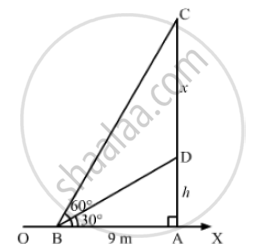# On a Horizonal Plane There is a Vertical Tower with a Flagpole on the Top of the Tower,Find the Height of the Tower and the Flagpole Mounted on It. - Mathematics

On a horizonal plane there is a vertical tower with a flagpole on the top of the tower. At a point, 9 meters away from the foot of the tower, the angle of elevation of the top and bottom of the flagpole are 60 and 30 respectively. Find the height of the tower and the flagpole mounted on it.

#### Solution

Let OX be the horizontal plane, AD be the tower and CD be the vertical flagpole
We have:

AB = 9m, ∠DBA= 30° and ∠CBA = 60°
Let:
AD = hm and CD = xmIn the right ΔABD,we have:

(AD)/(AB) = tan 30° = 1/ sqrt(3)

⇒ h/8 = 1/sqrt(3)

⇒ h = 9/sqrt(3) = 5.19 m

Now, in the right  ΔABC,we have

(AC)/(BA) = tan 60° = sqrt(3)

⇒  (h+x ) /9 = sqrt(3)

⇒  h + x = 9 sqrt(3)

By putting h= 9/sqrt(3) in the above equation, we get:

9/sqrt(3) + x = 9 sqrt(3)

⇒   x = 9 sqrt(3) - 9/ sqrt(3)

⇒  x = (27-9)/ sqrt(3) = 18/ sqrt(3) = 18/ 1.73 = 10.4

Thus, we have:
Height of the flagpole = 10. 4 m
Height of the tower = 5. 19 m

Is there an error in this question or solution?
Chapter 14: Height and Distance - Exercises

#### APPEARS IN

RS Aggarwal Secondary School Class 10 Maths
Chapter 14 Height and Distance
Exercises | Q 10

Share